### Home > PC > Chapter 6 > Lesson 6.1.1 > Problem6-18

6-18.
1. Solve each equation for x. Homework Help ✎

1.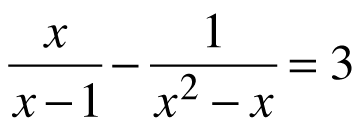2.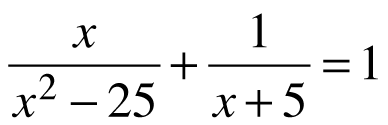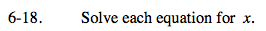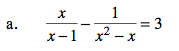Multiply each term by x(x − 1).
Simplify.
Solve.

$\frac{x(x-1)(x)}{x-1}-\frac{x(x-1)}{x(x-1)}=3x(x-1)$

x2 − 1 = 3x2 − 3x
2x2 − 3x + 1 = 0
(2x − 1)(x − 1) = 0

$x=\frac{1}{2}\text{ or }x\neq 1 \text{ undefined}$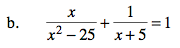Use a similar process as part (a).